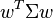# Adaptive Asset Allocation: minimum variance portfoliosThis is a continuation of my previous post on adaptive asset allocation.

Introduction to Mean-Variance Optimization

Mean-variance optimization (the implementation of Markowitz’s modern portfolio theory) basically allows one to find the optimal weights of assets in a portfolio that maximizes expected return given a level of risk/variance, or equivalently, minimize risk/variance given a level of expected return. The biggest ingredient in mean-variance optimization is the covariance matrix of the assets’ returns. The covariance matrix contains information on not only how volatile the assets are (their variance) but also how they move with each other (covariance). Covariance adds a piece to the adaptive asset allocation puzzle that we did not have before: how the asset classes move with each other, how correlated they are, if they’re good hedges for each other. The minimum variance portfolio is the set of asset class weights that minimizes the variance of the portfolio (regardless of our expectations of future returns).

This is a step up from risk parity, which assigns each asset class a weight such that all asset classes in the portfolio contribute the same amount of variance to the portfolio variance. The overall portfolio variance could still be relatively high. Now our portfolios are being optimized to have the smallest variance possible.

I won’t get too deep into the details of the math, but there is a closed form solution to finding the set of optimal portfolio weights. It’s minimizing the quadratic functionwhere

•$w$ is a vector of holding weights such that$sum w_i = 1$
•$Sigma$ is the covariance matrix of the returns of the assets
•$q ge 0$ is the “risk tolerance”:$q = 0$ works to minimize portfolio variance and$q = infty$works to maximize portfolio return
•$R$ is the vector of expected returns
•$w^{T} Sigma w$ is the variance of portfolio returns
•$R^{T} w$ is the expected return on the portfolio

For the minimum variance portfolio,$q = 0$ , so we’re actually just minimizing. Actual implementation was done with python’s cvxopt (convex optimization) library.

## Results

(like last time, all portfolios are rebalanced monthly)

Minimum Variance Portfolio

tl;dr: CAGR lower, max drawdown less severe, Sharpe Ratio slightly higher than that of the momentum + risk parity portfolio.

Where all ETFs are traded, and are weighted in the portfolio such that the variance of the portfolio is minimized.Momentum and Minimum Variance Portfolio

tl;dr: compared to the momentum + risk parity portfolio (highest Sharpe Ratio so far, talked about in the previous post): CAGR about the same, max drawdown less severe (what’s even more impressive is that the max drawdown during the recent financial crisis was only -13%), Sharpe Ratio slightly higher

Where only the top five ETFs are selected based on their momentum, and are weighted in the portfolio such that the variance of the portfolio is minimized.Summary

The last portfolio dominates all the other portfolios tested: it has the highest CAGR, smallest max drawdown, and highest Sharpe Ratio. This is because it includes all three pieces of the asset allocation puzzle: returns, variance, and correlation/covariance. We’ve made asset allocation more adaptive by filtering assets by momentum (with the expectation that high momentum assets will continue to perform well in the near term) and using shorter timeframes for variance and correlation/covariance–through this, the portfolios are more responsive to more recent asset price action.

## 8 thoughts on “Adaptive Asset Allocation: minimum variance portfolios”

1.arco2 says:

For research purpose, you should show as well the ~20 path generated by starting on another day of the month, as the monthly rebalance in conjunction with momentum has a very high path-dependency. Rebalance costs are not neglectable anymore, as the generated turnover is fairly high (in term of spread to pay for getting in/out). Happy tradingy.

1.Troy says:

That is a good point. I will take that into account, thank you for the feedback

2.noumann says:

Great work,but what do you mean for “shorter timeframes for variance and covariance Matrix”? 3,6,12 months or what else?
Thank you

1.Troy says:

I believe I chose 6 months

3.keyser1 says:

Could you make a blog post on minimal covariance?